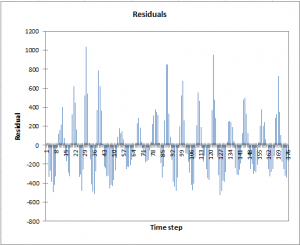# Suavización de series temporales

Smoothing of time series allows extracting a signal and forecasting future values. Several methods available in Excel using the XLSTAT statistical software.Several smoothing methods are available in the XLSTAT-Forecast solution. They are described below.

## Simple exponential smoothing

This model is sometimes referred to as Brown's Simple Exponential Smoothing, or the exponentially weighted moving average model. Exponential smoothing is useful when one needs to model a value by simply taking into account past observations. It is called "exponential" because the weight of past observations decreases exponentially. This method it is not very satisfactory in terms of prediction, as the predictions are constant after n+1.

## Double exponential smoothing

This model is sometimes referred to as Brown's Linear Exponential Smoothing or Brown's Double Exponential Smoothing. It allows taking into account a trend that varies with time. The predictions take into account the trend as it is for the last observed data.

## Holt’s linear exponential smoothing

This model is sometimes referred to as the Holt-Winters non seasonal algorithm. It enables taking into account a permanent component and a trend that varies with time. This model adapts itself quicker to the data compared with the double exponential smoothing. It involves a second parameter. The predictions for t>n take into account the permanent component and the trend component.

This method considers a trend that varies with time and a seasonal component with a period p. The predictions take into account the trend and the seasonality. The model is called additive because the seasonality effect is stable and does not grow with time.

## Holt-Winters seasonal multiplicative model

This method ponders a trend that varies with time and a seasonal component with a period p. The predictions take into account the trend and the seasonality. The model is called multiplicative because the seasonality effect varies with time. The more the discrepancies between the observations are high, the more the seasonal component increases.

Note 1: for all the above models, XLSTAT estimates the values of the parameters that minimize the mean square error (MSE). However, it is also possible to maximize the likelihood, as, apart from the Holt-Winters multiplicative model, it is possible to write these models as ARIMA models. For example, the simple exponential smoothing is equivalent to an ARIMA(0,1,1) model, and the Holt-Winters additive model is equivalent to an ARIMA (0,1,p+1)(0,1,0) p. If you prefer to maximize the likelihood, we advise you to use the ARIMA procedure of XLSTAT.

Note 2: for all the above models, initial values for S, T and D, are required. XLSTAT offers several options, including backcasting to set these values. When backcasting is selected, the algorithm reverses the series, starts with simple initial values corresponding to the Y(x) option, then computes estimates and uses these estimates as initial values.

## Moving average

This model is a simple way to take into account past and optionally future observations to predict values. It works as a filter that is able to remove noise. While with the smoothing methods defined below, an observation influences all future predictions (even if the decay is exponential), in the case of the moving average the memory is limited to q. If the constant l is set to zero, the prediction depends on the past q values and on the current value, and if l is set to one, it also depends on the next q values. Moving averages are often used as filters, and not as way to do accurate predictions.

## Fourier smoothing

The concept of the Fourier smoothing is to transform a time series into its Fourier coordinates, then remove part of the higher frequencies, and then transform the coordinates back to a signal. This new signal is a smoothed series.### analice sus datos con xlstat

prueba gratuita de 14 días

Incluido en